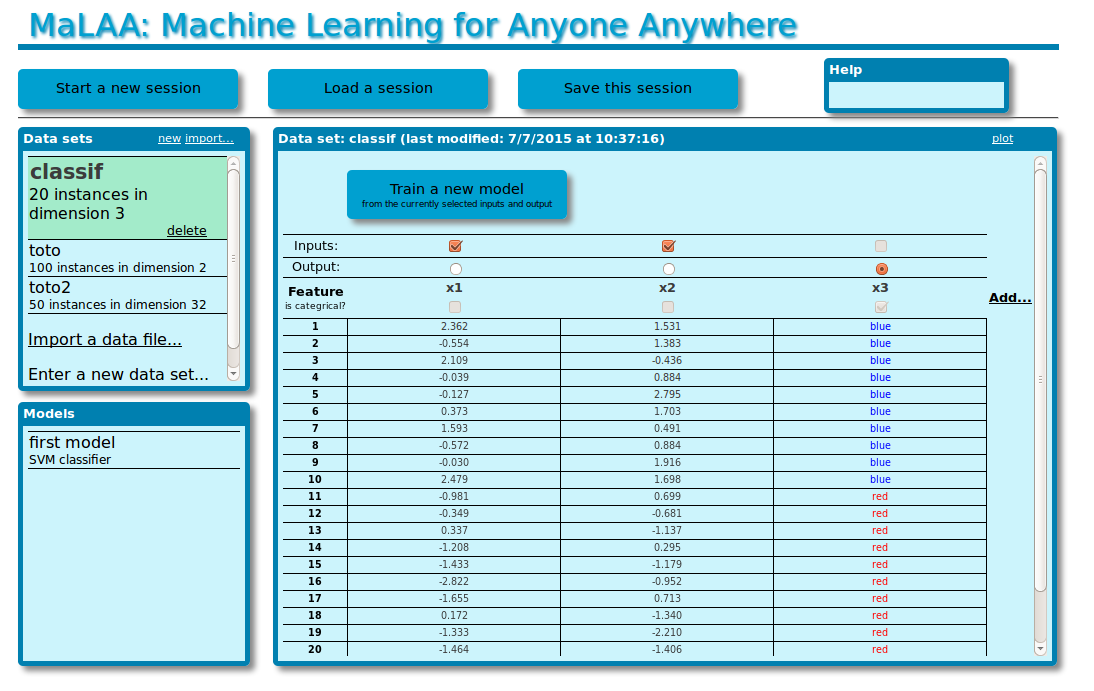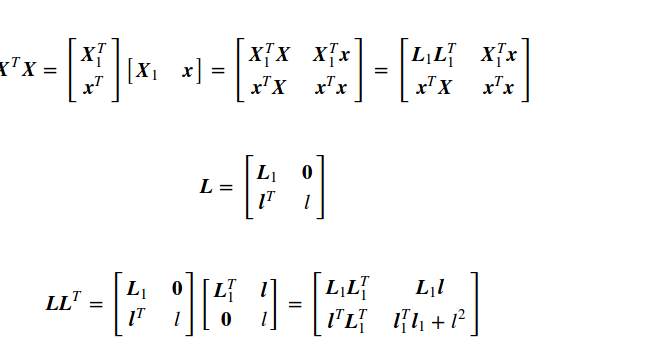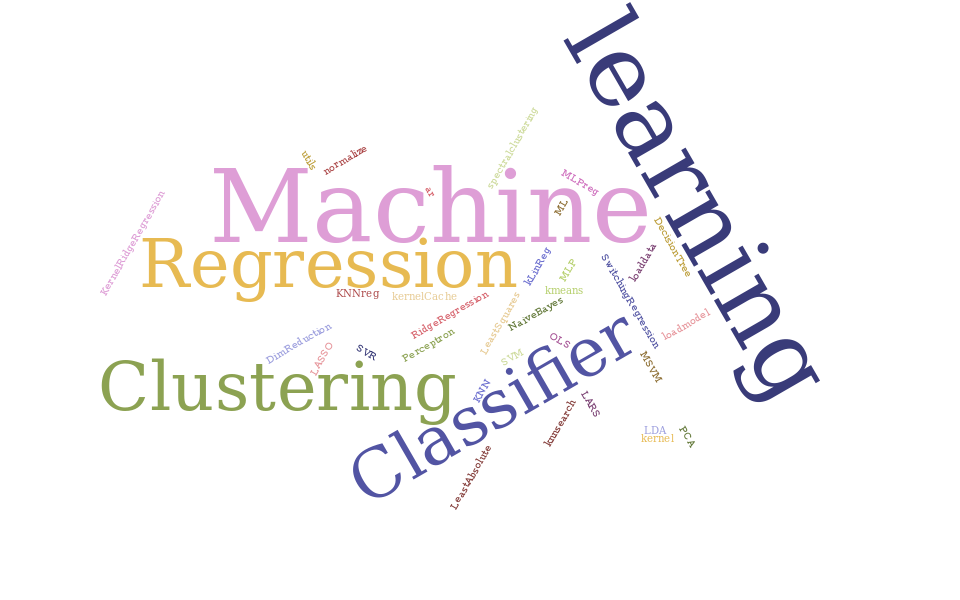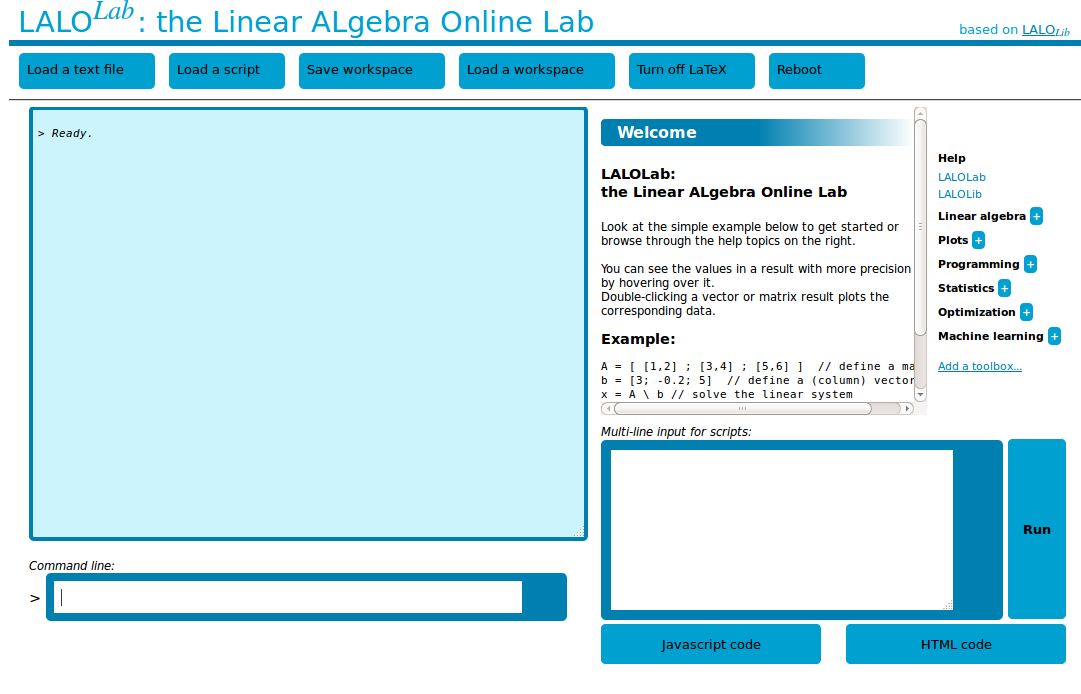## MaLAA

Machine Learning for Anyone Anywhere

MaLAA is a simple web application built with MLweb and aimed at providing anyone with access to state-of-the-art machine learning tools for performing prediction tasks in a fully automated manner.

No need to be an expert of machine learning or computer science. Simply upload your data and ask for predictions. Privacy is warranted: nothing leaves your computer.

Next``````
X = rand(60,30);
singularvalues = svd(X);
KyFan3 = sum(get(singularvalues, range(3)));
``````
``````
A = randn(1000,100);
x = randn(100);
y = add( mul(A,x), mul(0.01, randn(1000)) );
xhat = solve(A, y);
``````

## LALOLib

Scientific computing in web pages made easy

LALOLib (Linear ALgebra Online Library) is a library that enables and ease scientific computing in web pages or applications. It includes functions for basic linear algebra, statistics and optimization, all easily loaded with a simple line of HTML: `<script src="http://mlweb.loria.fr/lalolib.js"></script>`

Nothing to run on the server-side, nothing to setup on the client-side. Just plain javascript computations that work out of the box.

Next``````
svm = new Classifier(SVM, {kernel: "rbf"});
trainingError = svm.train(X,Y);
labels = svm.predict(Xtest);
``````
``````
model = new Regression(RidgeRegression, {lambda: 0.1});
mse = model.train(X,y);
yhat = model.predict( x );
``````

### List of algorithms

Classification: K-nearest neighbors, Linear discriminant analysis, Naive Bayes classifier, Perceptron, Multi-layer perceptron, Support vector machines, Multi-class support vector machines, Decision trees
Regression: Least squares, Least absolute devations, K-nearest neighbors, Ridge regression, LASSO, LARS, Orthogonal least squares, Multi-layer perceptron, Kernel ridge regression, Support vector regression, K-LinReg
Clustering: K-means, Spectral clustering
Dimensionality reduction: Principal component analysis, Locally linear embedding, Local tangent space alignment

## ML.js

Extending web applications with machine learning capabilities

ML.js extends LALOLib with easy-to-use functions implementing the state of the art in machine learning for classification, regression and clustering.

With ML.js, a web page can for instance train a support vector machine on the fly and deliver predictions in complete privacy without communicating with a distant server.

Load ML.js with the HTML line `<script src="http://mlweb.loria.fr/ml.js"></script>`

Next``````
A = [ [1,2] ; [3,4] ; [5,6] ]  // define a matrix
b = [3; -0.2; 5]  // define a (column) vector
x = A \ b // solve the linear system
plot( A*x - b )  // plot the error
``````

## LALOLab

An online laboratory for scientific computations

LALOLab is an online numerical computing environment is the flavor of Matlab that offers a high-level language to ease the development of scientific and machine learning web applications based on LALOLib and ML.js.

LALOLab comes with an online help of LALOLib and ML.js functions including many examples and can easily be extended with more toolboxes.

Next

MLweb is still under development and is provided without warranty.

### Scientific background

Learn more about machine learning and the scientific foundations of MLweb in the webbook An interactive journey into machine learning.

### Efficiency and responsiveness

LALOLib and MLweb were designed to maximize efficiency. Yet, machine learning computations can take a lot of time. In such cases, responsiveness can be maintained by using the libraries in asynchronous mode, as explained here.

### Privacy

With MLweb, all computations are performed on the client side: the data never travels across the internet and never gets stored on a server.

### Extendability

MLweb can be easily extended with new machine learning functions or other toolboxes. Learn how to do this here.

### Credits

MLweb is an open-source project conducted by F. Lauer at the LORIA, and funded by the University of Lorraine, France.

If you use MLweb in research work, please cite the following paper:
F. Lauer. MLweb: a toolkit for machine learning on the web. Neurocomputing, 282:74-77, 2018.